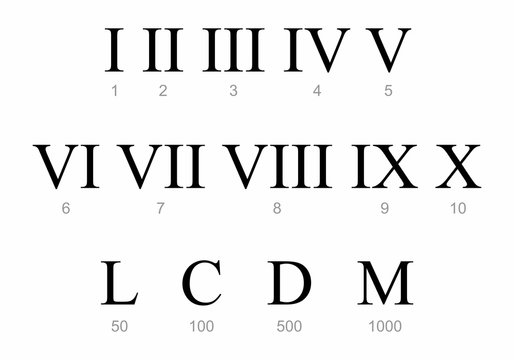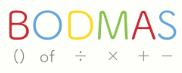Courses

# Roman Numerals Class 6 Notes | EduRev

## Class 6 : Roman Numerals Class 6 Notes | EduRev

The document Roman Numerals Class 6 Notes | EduRev is a part of the Class 6 Course Mathematics (Maths) Class 6.
All you need of Class 6 at this link: Class 6

Look at the bottom of the cover page of your textbook. It says-‘ Textbook for class VI’. This numeral VI is 6 in the Hindu-Arabic system. Likewise, if we look around we can spot various Roman numerals. Many watches have numbers written in Roman numerals. Thus although Hindu-Arabic numerals are most widely used, the Roman numerals often appear in our daily lives.

Some basic Roman numerals areFew Roman numerals

1→ I             10→X             C→100
2→ II           20→XX         D→500
3→ III         30→XXX      M→1000
4→ IV         40→XL
5→ V           50→L
6→VI          60→LX
7→VII         70→LXX
8→VIII       80→LXXX
9→IX          90→XC
Rules of the Roman Numeral System

Roman numbers follow certain rules, which helps determine how the number is written. It will also help us in reading or recognizing roman numbers. Let us take a look.

1. If a symbol is repeated, its value is added as many times as it occurs.
Example: II = 2, XX = 20 etc.
2. The symbols V, L and D are never repeated.
3. A symbol is not repeated more than three times.
4. If a smaller symbol is written to the right of a symbol with a greater value, then its value gets added to the value of the greater symbol.
Example: VI = 5+1 = 6, XI = 11 and so on.
5. If a smaller symbol is written on the left of a symbol with a greater value, then its value is subtracted from the value of the greater symbol.
Example: IV = 5-1 = 4, IX = 9 etc.
6. The symbols V, L and D are never written to the left of a symbol of greater value i.e. V, L and D are never subtracted. The symbol I can be subtracted from V and X only. The symbol X can be subtracted from L, M and C only.
7. A symbol cannot be subtracted more than once from a particular symbol of greater value. In other words, we cannot repeat a symbol on the left side of a symbol. Eg, 98 is written as XCVIII and not as IIC.

Solved Examples

Ques 1: Convert the following numerals to the Roman numerals:
a. 69
b. 98
c. 109
d. 786
e. 1298
f. 2018
Ans: a. 69 = 60 + 9 = (50+10) + 9 = LX + IX = LXIX
b. 98 = 90+8 = (100-10) + 8 = XC + VIII = XCVIII
c. 109 = 100 + 9 = C + IX = CIX
d. 786 = 700 + 86 = (500 + 200) + ( 80 + 6) = DCC + LXXXVI = DCCLXXXVI
e. 1298 = (1000 + 200) + (90 + 8) = MCC + [(100-10) + 8)] = MCC + XCVIII = MCCXCVIII
f. 2018 = 2000 + 18 = MM + XVII= MMXVII

Ques 2: Convert 1984 to Roman Numerals
Ans: We can write 1984 as 1000+900+80+4
Then 1000 = M
900 = CM
80 = LXXX
4 = IV
Hence, 1984 in Roman Letters is MCMLXXXIV

BODMAS Rule

Use of Brackets

First, we must understand the use of brackets in mathematics. It is a very important part of forming and solving equations. Let us take an example. You went to the store to buy chocolates. You went a little overboard and bought ten chocolates. Two of them cost Rs. 10/- each, seven of them were Rs 5/- each and one was for Rs 30/-. Now how will you represent this mathematically?

Well the equation will go something like this

2 × 10 + 5 × 7 + 1 × 30 = 85

However doesn’t the above equation seem very confusing? And if someone was asked to solve it he would be prone to errors and miscalculations. So let us conjure a better way of forming this equation using brackets.

(2 × 10) + (5 × 7) + (1 × 30) = 85

Doesn’t this make much more sense? It is an organized and systematic representation of the scenario. And the person solving the equation will have a better understanding as well. So you can see how the use of brackets is quite essential in mathematics.

BODMASNow moving on to the BODMAS rule. In the above equation, we saw that we were performing two operations, namely addition and multiplication. But imagine that in an equation you had to perform more such operations. It would be confusing. And which operation will you perform first? Take for example the following equation.
23 × 7 + 78 ÷ 2 = ?
So how do you solve such a problem? This is where the BODMAS rule comes in. It gives us an order in which to perform the mathematical operations in a given problem or equation.
It is actually an acronym and goes as follows

• B: Brackets
• O: Of
• D: Division
• M: Multiplication
• S: Subtraction

This is the order in which you perform the operations to arrive at the correct answer. You first solve the operations within the bracket. This is called “opening the bracket’. Then you move on to division, after which you perform multiplication if the equation requires it from left to right. And finally, you add the numbers and lastly perform the subtraction.
The best way to understand the BODMAS rule is through examples. So let us see a few problems and solve them using BODMAS.

Solved Examples
Ques 1: Solve and find the value of x using the BODMAS rule.
42 ÷ 2 + x × 3 − 22 = 8
a. 2
b. 5
c. 4
d. 3
Sol: 42÷2+x×3−22 = 8
⇒ 21 + 3 × x = 8 +22 = 30 (Using BODMAS rule)
⇒ 3 × x =30 − 21 = 9
⇒ x = 9/3 = 3

Ques 2: Simplify 3+3 of 3÷3 of 3×3.
Sol: Applying the BODMAS rule,
3+3 of 3÷3 of 3×3
= 3+(3 of 3)÷3 of 3×3
= 3+9÷3 of 3×3
= 3+3 of 3×3
= 3+(3 of 3)×3
= 3+9×3
= 3+(9×3)
= 3+27 = 30

Ques 3: In the given equation find which of the following interchange of signs/numbers would make the equation correct 9 + 7 × 1= 5 − 3
a. + with –
b. + with ×
c. × with –
d. None of the above
Sol: The correct option is “C”.
We substitute × on the place of − and − on the place of × then the equation becomes correct
9 + 7 − 1 = 5 × 3
16 − 1 = 15
15 = 15
That is true.

Offer running on EduRev: Apply code STAYHOME200 to get INR 200 off on our premium plan EduRev Infinity!

## Mathematics (Maths) Class 6

185 videos|229 docs|43 tests

,

,

,

,

,

,

,

,

,

,

,

,

,

,

,

,

,

,

,

,

,

;